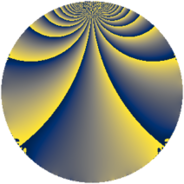# Properties

 Label 273.2.gLevel $273$ Weight $2$ Character orbit 273.g Rep. character $\chi_{273}(272,\cdot)$ Character field $\Q$ Dimension $32$ Newform subspaces $1$ Sturm bound $74$ Trace bound $0$

# Related objects

## Defining parameters

 Level: $$N$$ $$=$$ $$273 = 3 \cdot 7 \cdot 13$$ Weight: $$k$$ $$=$$ $$2$$ Character orbit: $$[\chi]$$ $$=$$ 273.g (of order $$2$$ and degree $$1$$) Character conductor: $$\operatorname{cond}(\chi)$$ $$=$$ $$273$$ Character field: $$\Q$$ Newform subspaces: $$1$$ Sturm bound: $$74$$ Trace bound: $$0$$

## Dimensions

The following table gives the dimensions of various subspaces of $$M_{2}(273, [\chi])$$.

Total New Old
Modular forms 40 40 0
Cusp forms 32 32 0
Eisenstein series 8 8 0

## Trace form

 $$32q + 16q^{4} + O(q^{10})$$ $$32q + 16q^{4} - 16q^{16} - 16q^{25} + 16q^{30} - 32q^{36} - 48q^{42} + 48q^{43} - 32q^{49} - 16q^{51} - 80q^{64} + 32q^{78} + 80q^{79} - 48q^{81} - 96q^{88} + 32q^{91} + O(q^{100})$$

## Decomposition of $$S_{2}^{\mathrm{new}}(273, [\chi])$$ into newform subspaces

Label Dim. $$A$$ Field CM Traces $q$-expansion
$$a_2$$ $$a_3$$ $$a_5$$ $$a_7$$
273.2.g.a $$32$$ $$2.180$$ None $$0$$ $$0$$ $$0$$ $$0$$